Splitknockoff

library(SplitKnockoff)

Vignette for Splitknockoff

Author : Haoxue Wang, Yang Cao, Xinwei Sun, Yuan Yao

Introduction

Split Knockoff is a novel method for controlling the false discovery rate (FDR) in structural sparsity setting. This proposed scheme relaxes the linear subspace constraint to its neighborhood, often known as variable splitting in optimization. This vignette illustrates the usage of Splitknockoff with simulation experiments and will help you apply the split knockoff method in a light way. On top of that, an application to Alzheimer’s Disease study with MRI data demonstrates that the split knockoff method can disclose important lesion regions in brains associated with the disease and connections between neighboring regions of high contrast variations during disease progression.

install.packages("SplitKnockoff")   # just one line code to install our package

There is another Matlab package available. Simulation experiment of both packages verify the conclusion of the paper. This R package is more convenient when applied to new dataset as glmnet can be used directly by

install.packages("glmnet'")  # just one line code to install the glmnet tool

Key function

splitknockoff.filter(X, D, y, option) : Split Knockoff filter for structural sparsity problem.

splitknockoff.select(W, q): this function is for variable selection based on W statistics.

function involved frequently

private.hittingpoint(coef, lambda_vec): calculate the hitting time and the sign of respective variable in a path.

simu_unit(n, p, D, A, c, k, option): the simulation unit for simulation experiments.

split_knockoffs.create(X, y, D, nu, option): create a knockoff copy for A_gamma

split_knockoffs.statistics.pathorder.W_path(X, D, y, nu, option): generate the knockoff statistics W for beta from a split LASSO path in the intercepetion assignment step, using the method of path order.

Simulation Results

Figure 1b of the paper, comparing the different distributions of Knockoff statistics and Split Knockoff statistics.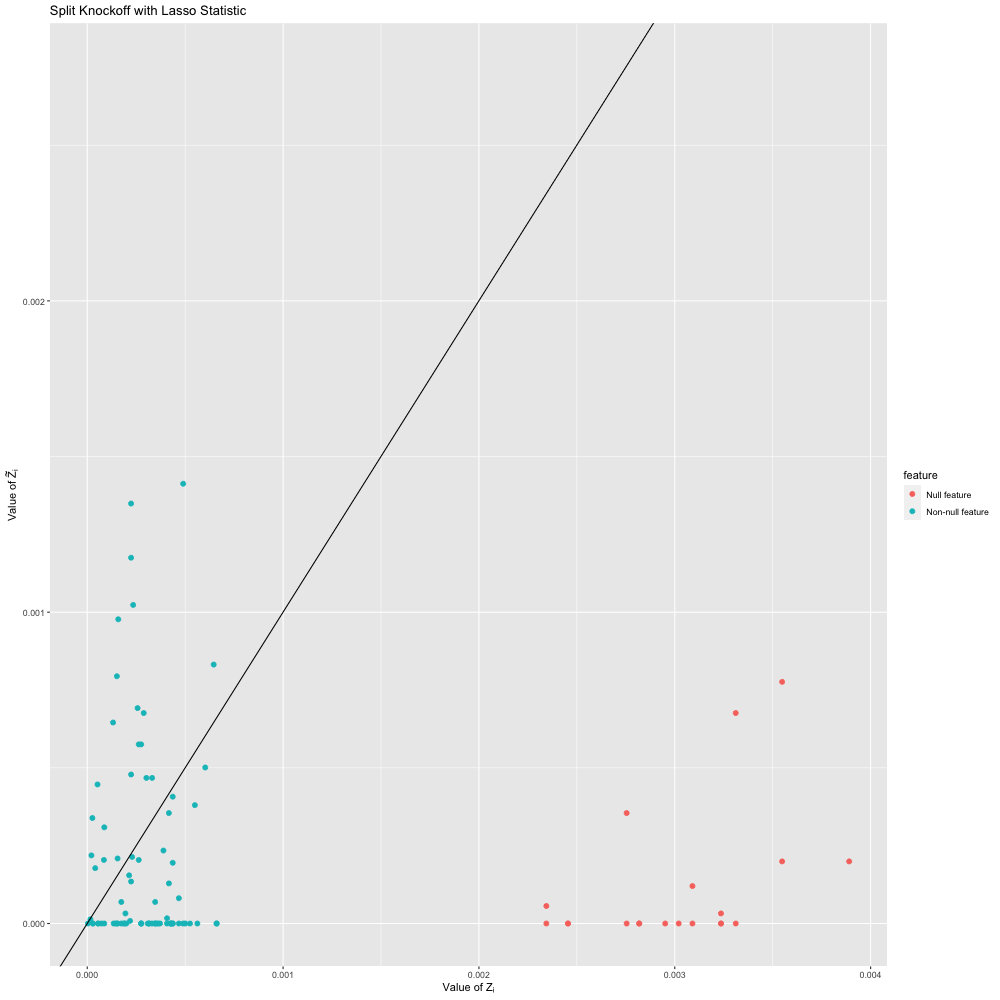Figure 2a of the paper, FDR in \(D_1\)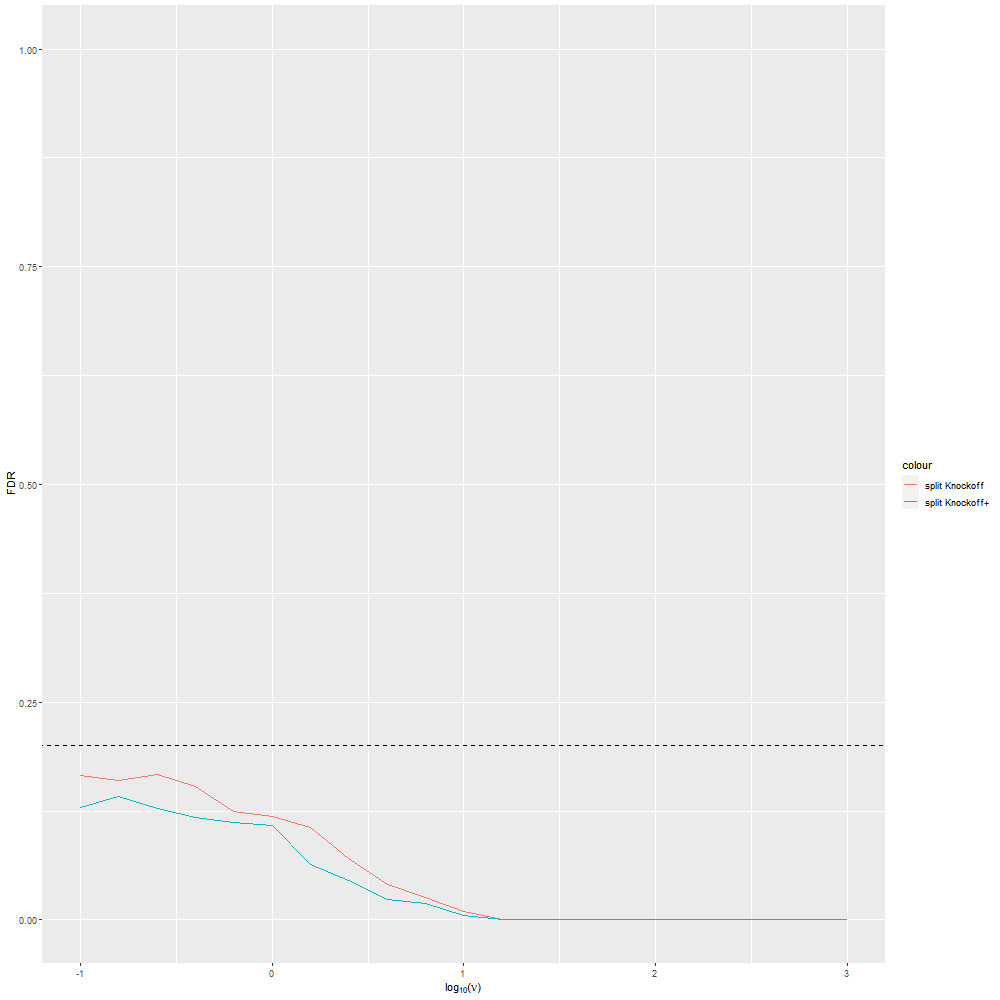Figure 2b of the paper, Power in \(D_1\)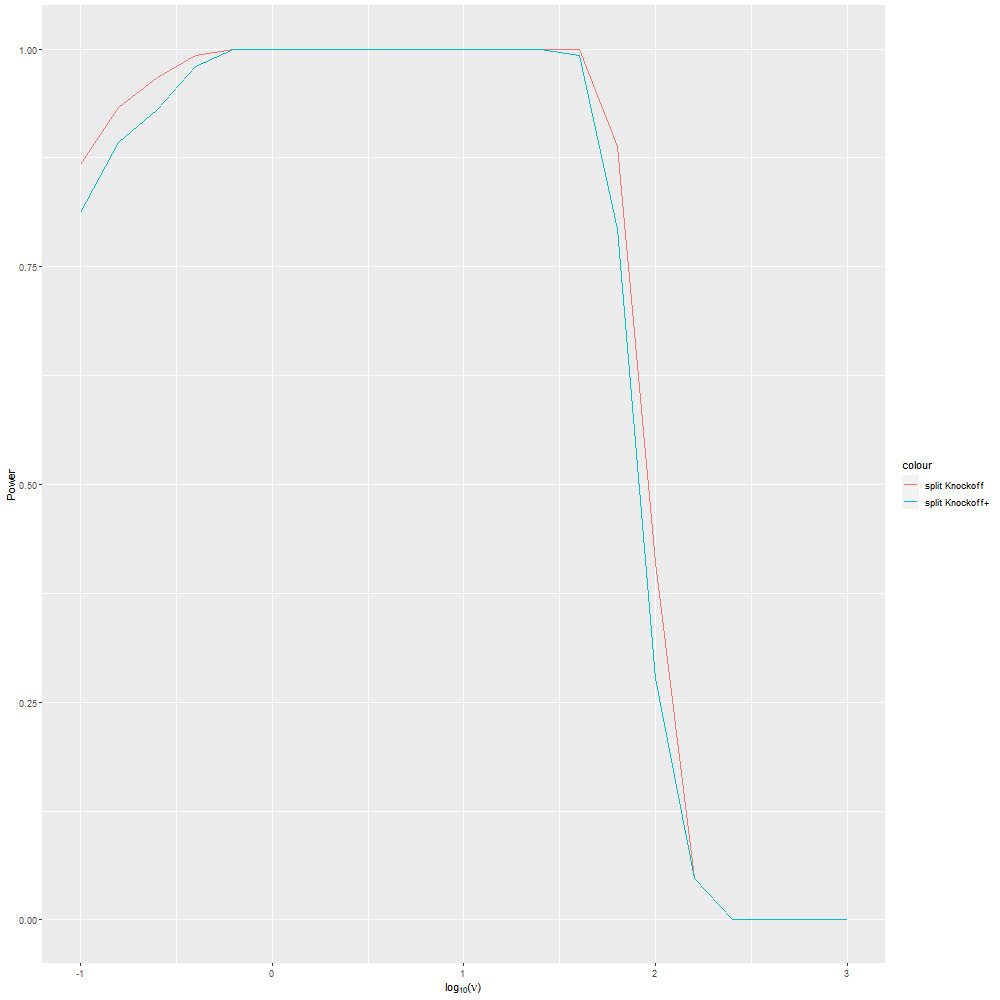Figure 2b of the paper, FDR in \(D_2\)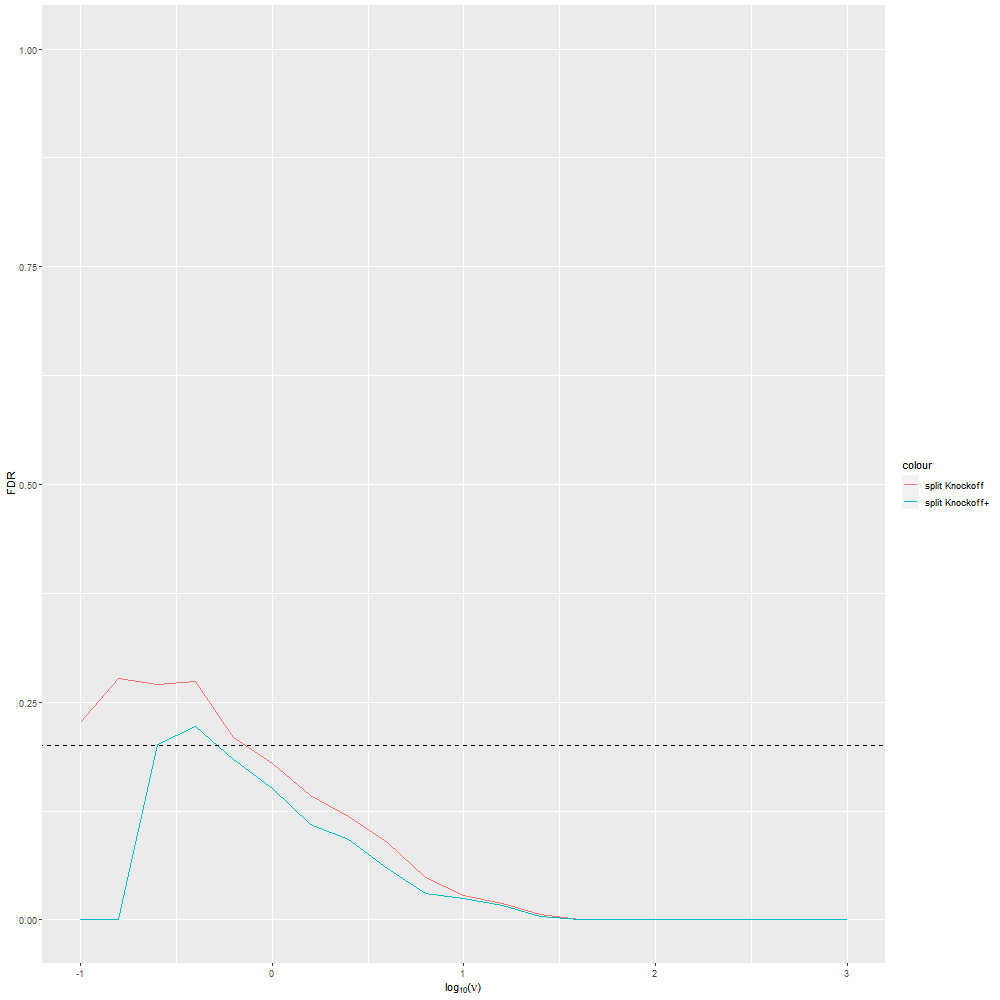Figure 2b of the paper, Power in \(D_2\)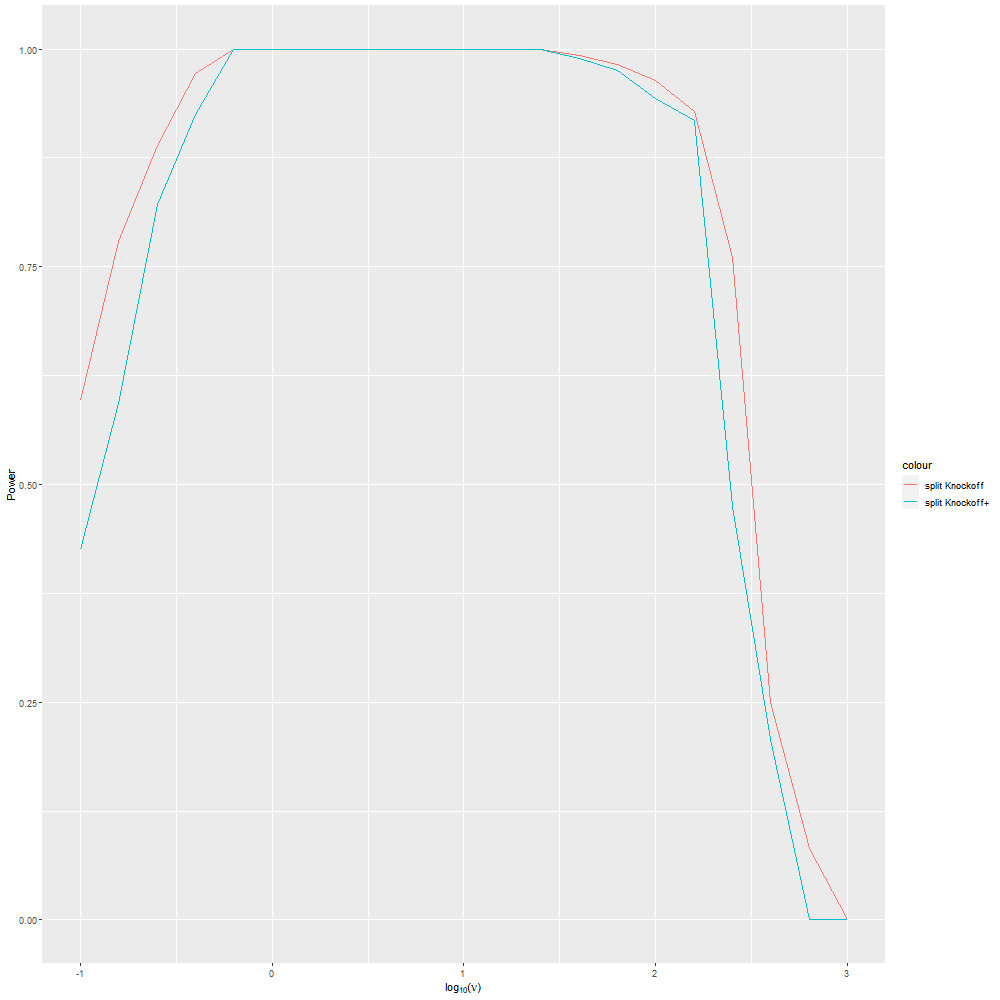Figure 2b of the paper, FDR in \(D_3\)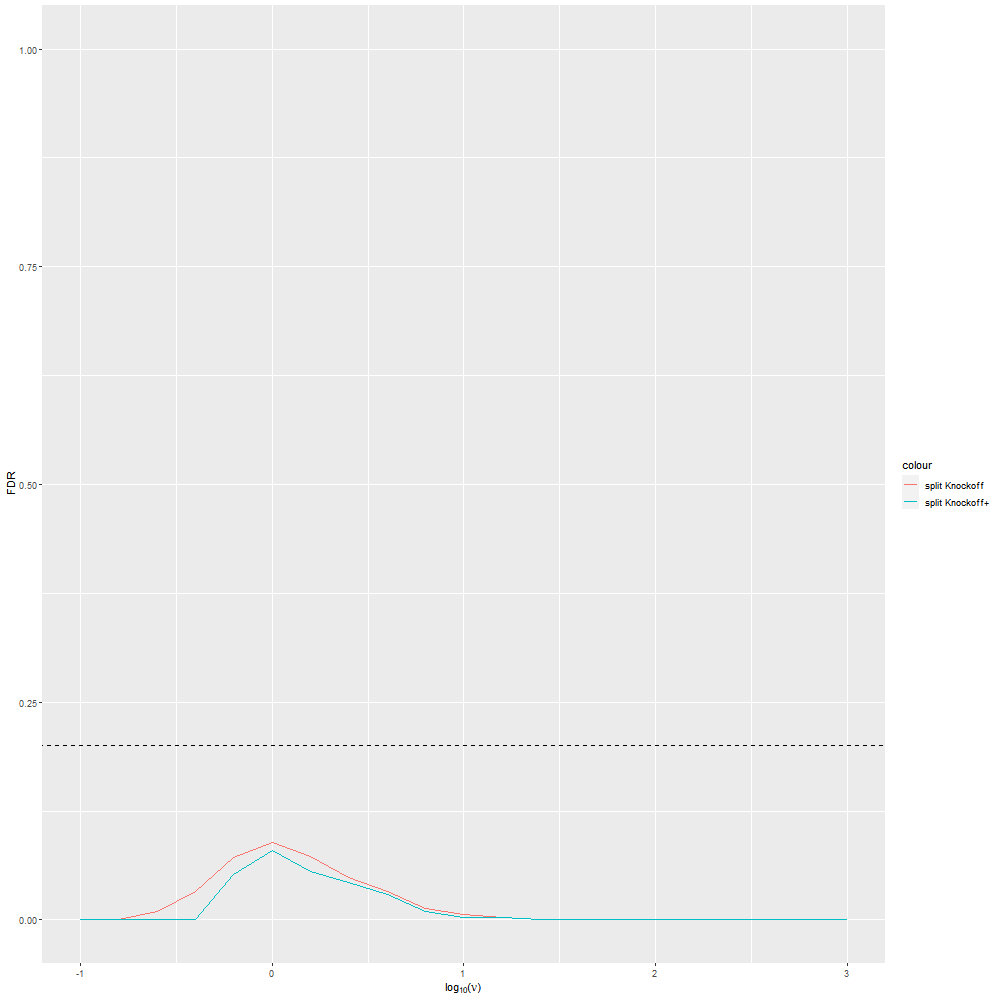Figure 2b of the paper, Power in \(D_3\)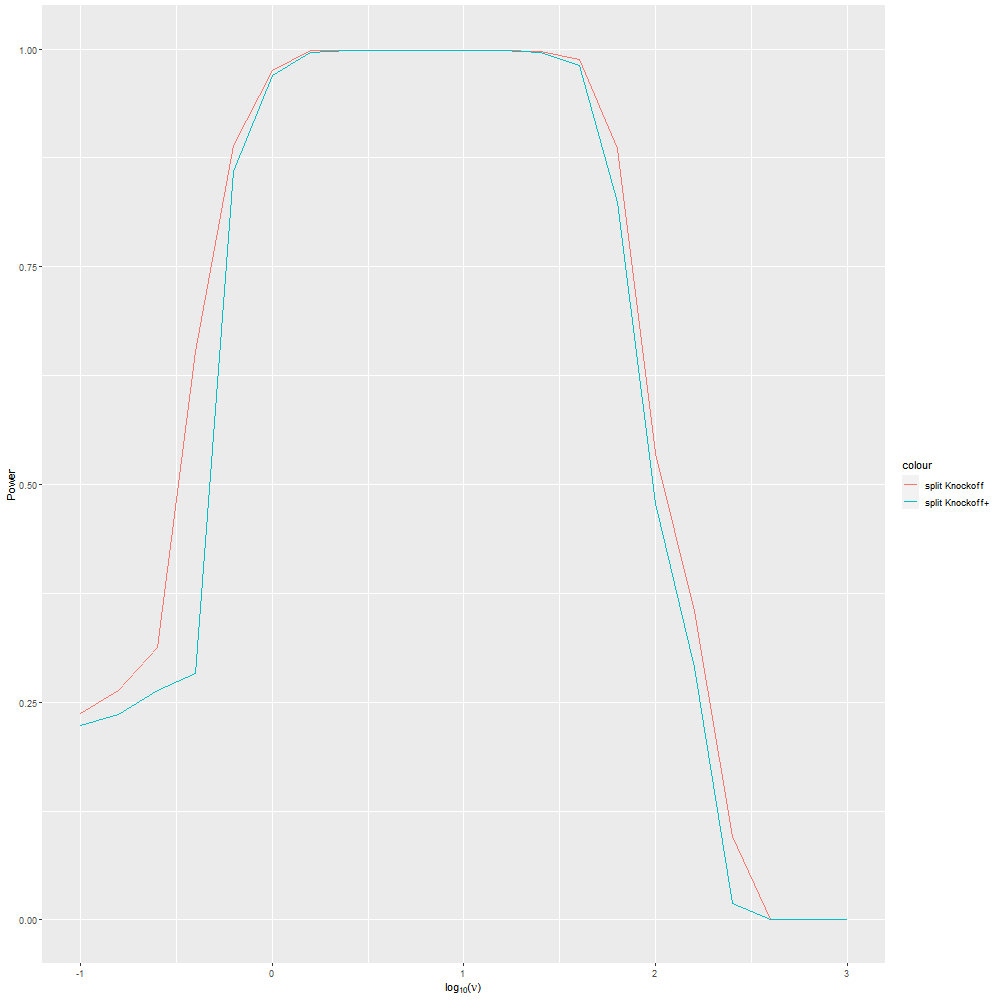Figure 3a of the paper, Effect of Feature Correlation: FDR for q = 0.2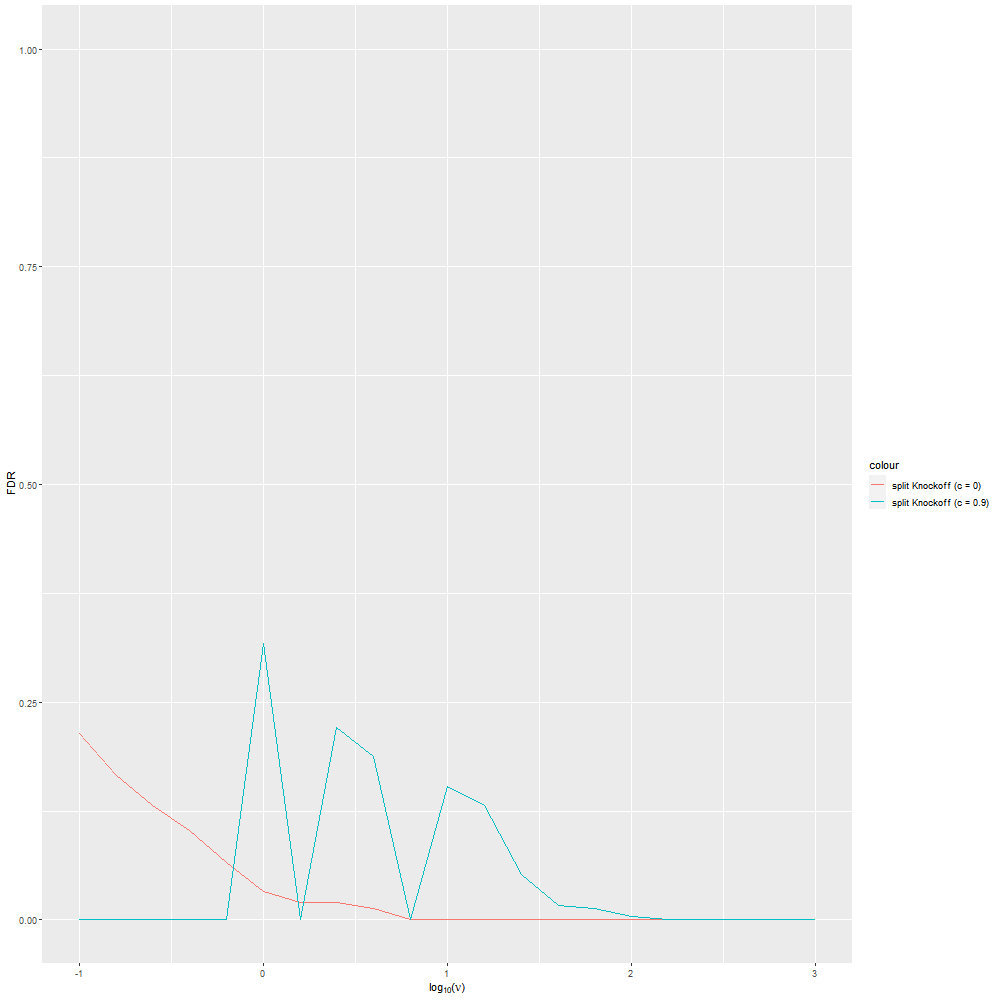Figure 3b of the paper, Effect of Feature Correlation: Power for q = 0.2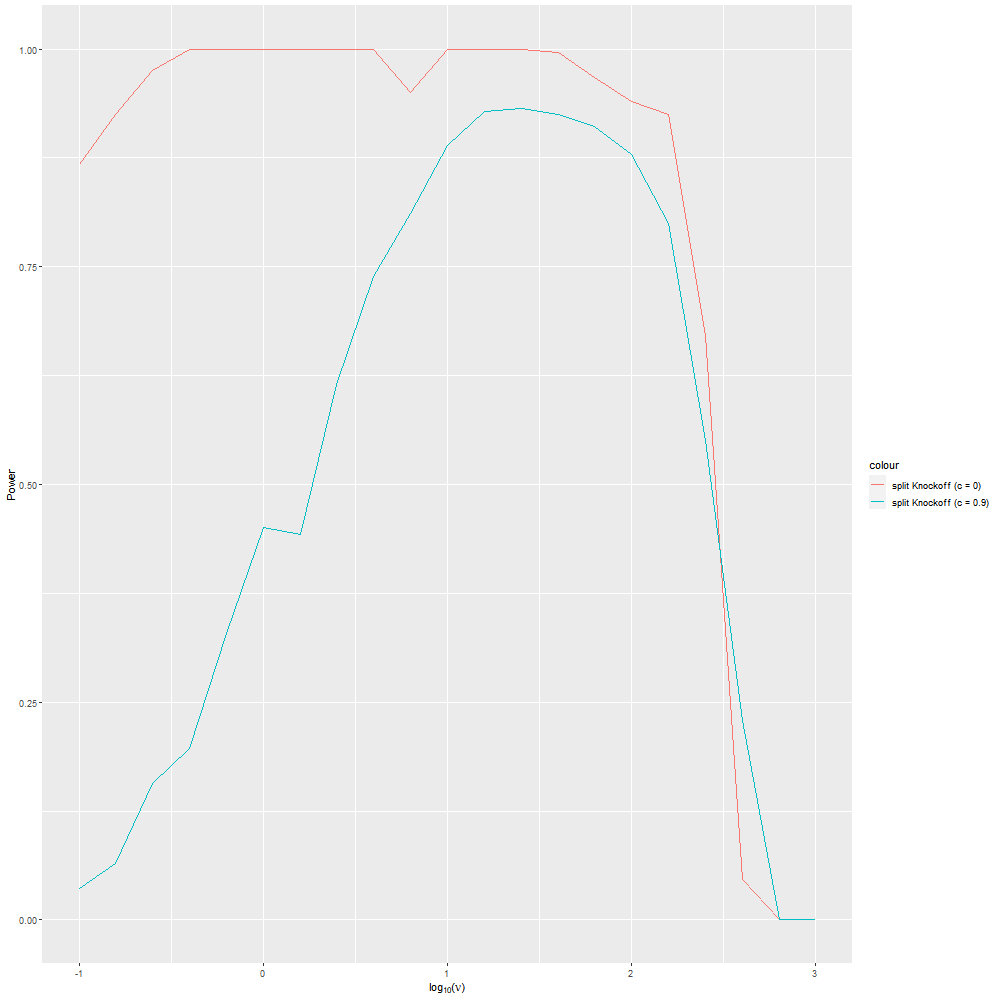Figure 4a of the paper, Effect of SNR: FDR for q = 0.2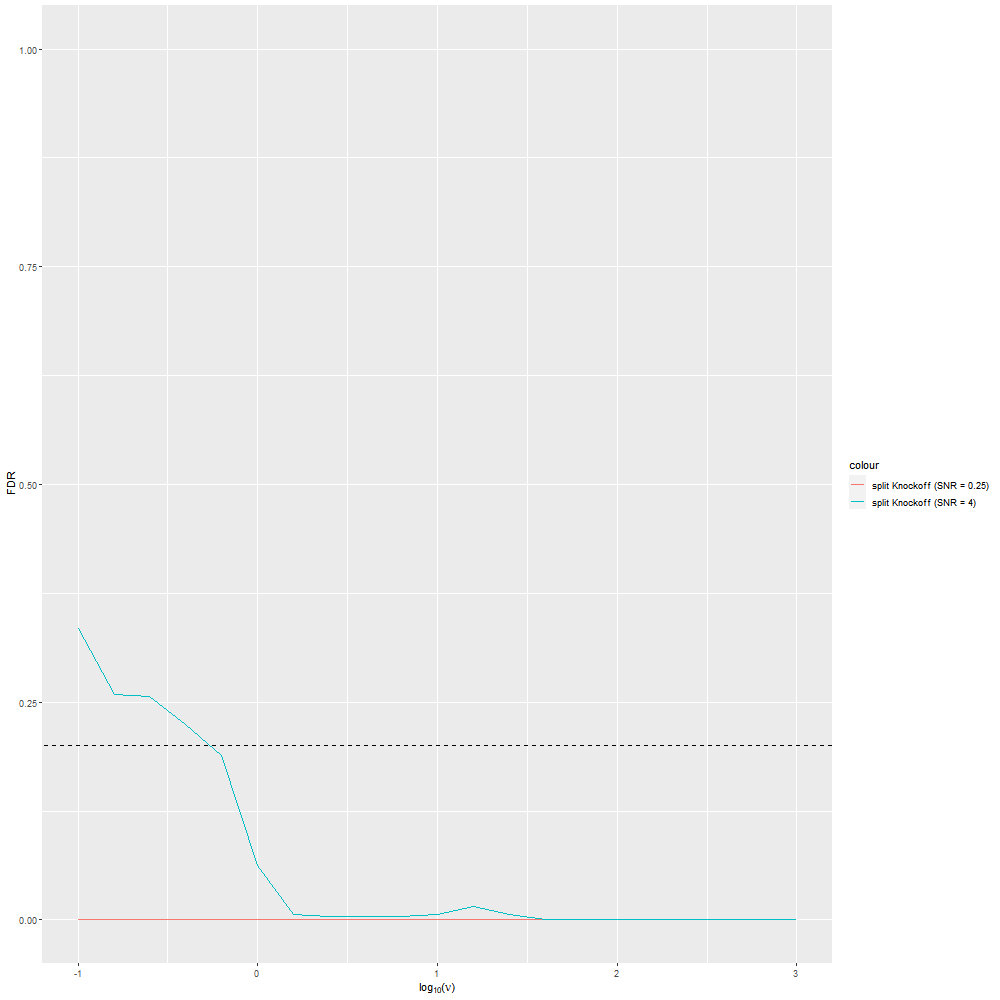Figure 4b of the paper, Effect of SNR: Power for q = 0.2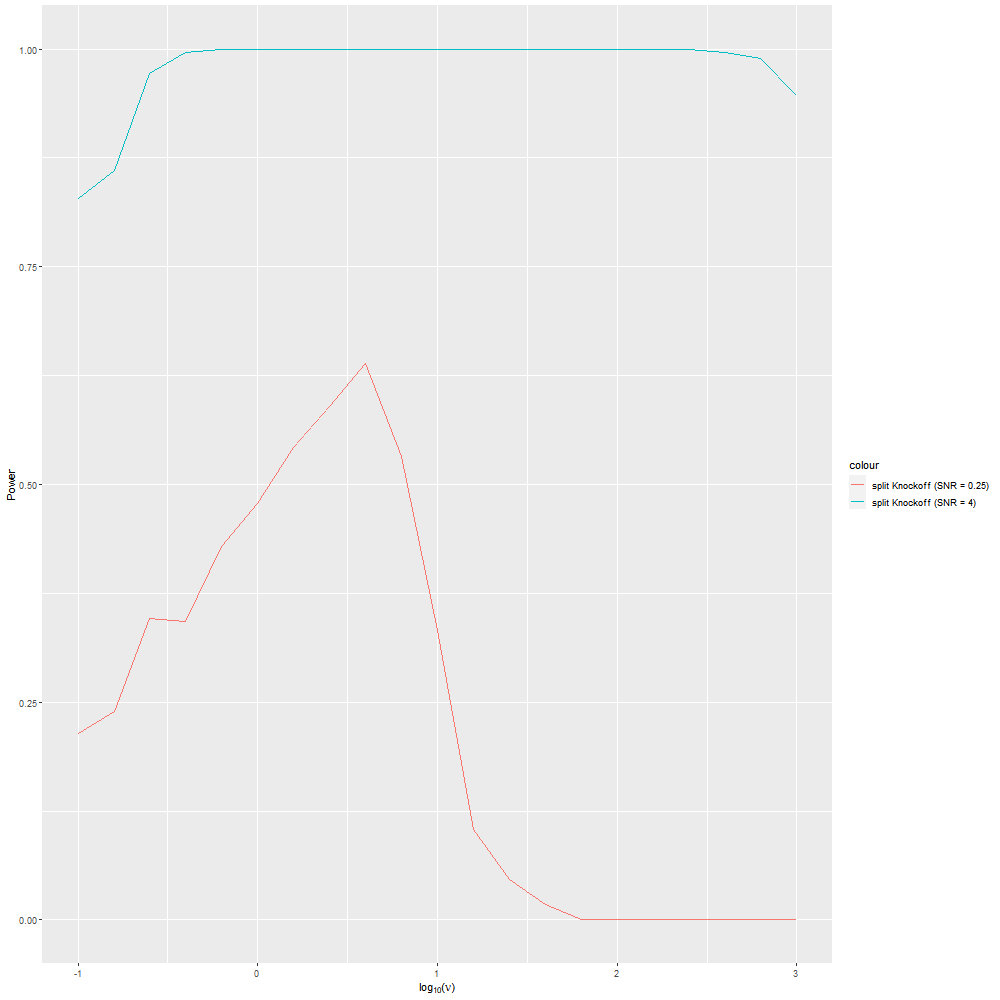Figure 5a of the paper, Effect of Sparsity Level: FDR for q = 0.2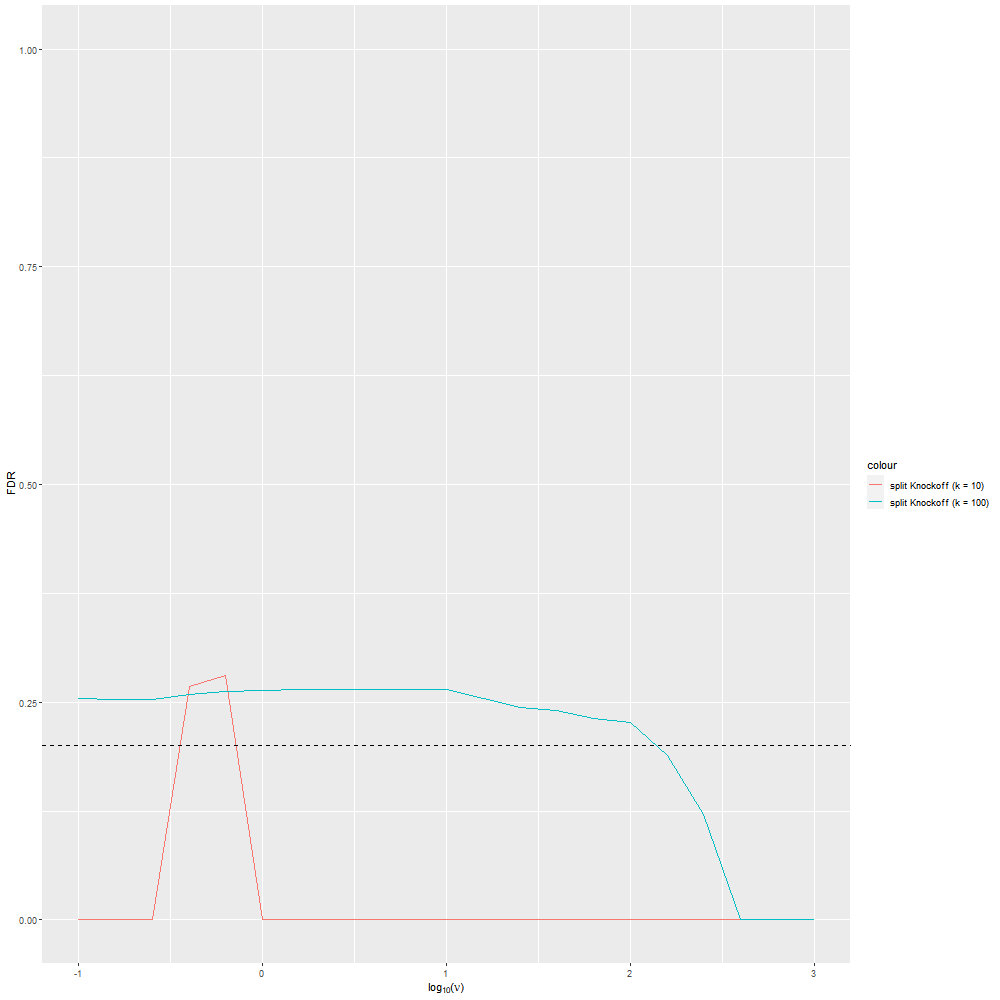Figure 5b of the paper, Effect of Sparsity Level: Power and FDR for q = 0.2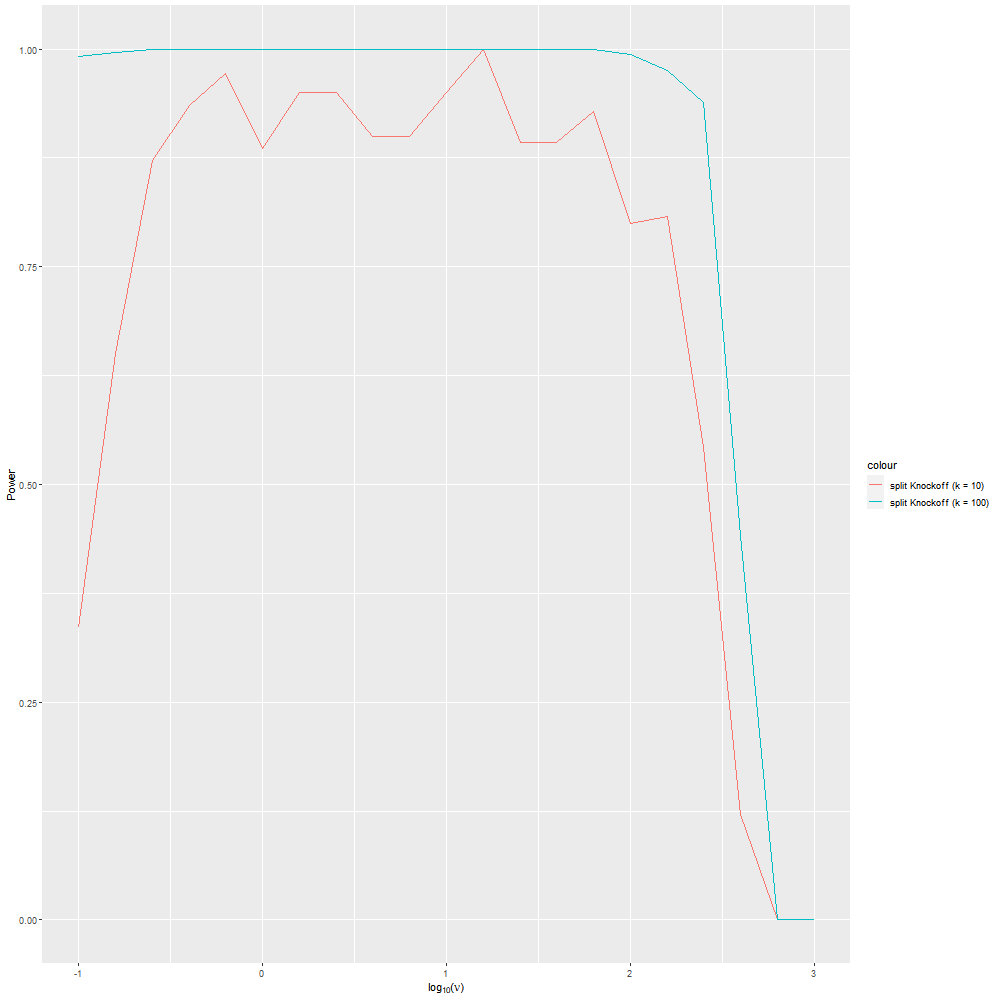For a complete reproduction of the simulation, you can see the code as fellow.

simulation details

Install all the packages and library them.
install.packages("SplitKnockoff")   # install our package

library(latex2exp)
library(ggplot2)
library(Matrix)
library(glmnet)
library(MASS)
library(SplitKnockoff)
set the parameter for the simulation
k <- 20   # sparsity level
A <- 1    # magnitude
n <- 350  # sample size
p <- 100  # dimension of variables
c <- 0.5  # feature correlation
sigma <-1 # noise level

option <- array(data = NA, dim = length(data), dimnames = NULL)
option\$q <- 0.2
option\$eta <- 0.1
option\$method <- 'knockoff'
option\$stage0 <- 'path'
option\$normalize <- 'true'
option\$cv_rule <- 'min'
option\$lambda <- 10.^seq(0, -6, by=-0.01)
option\$nu <- 10
option\$copy <- 'true'
option <- option[-1]
generate D and X
D <- diag(p)
m <- nrow(D)

# generate X
Sigma = matrix(0, p, p)
for( i in 1: p){
for(j in 1: p){
Sigma[i, j] <- c^(abs(i - j))
}
}

library(mvtnorm) # package mvtnorm needed for this generation
set.seed(100)
X <- rmvnorm(n,matrix(0, p, 1), Sigma) # generate X
generate beta and gamma
beta_true <- matrix(0, p, 1)
for( i in 1: k){
beta_true[i, 1] = A
if ( i%%3 == 1){
beta_true[i, 1] = -A
}
}
gamma_true <- D %*% beta_true

S0 <- which(gamma_true!=0)

generate varepsilon and noise and y

# generate varepsilon
set.seed(1)

# generate noise and y
varepsilon <- rnorm(n) * sqrt(sigma)
y <- X %*% beta_true + varepsilon

key step

use the key function filter to get the Z_path and t_Z_path

## Split Knockoff
filter_result <- splitknockoff.filter(X, D, y, option)
Z_path <- filter_result\$Z
t_Z_path <- filter_result\$t_Z

plot the figure

a <- sub("TRUE","0",is.element(1:m, S0))
b <- sub("FALSE","1",a)
df <- data.frame(x=Z_path[],y= t_Z_path[], feature=b)
save.image("C:/Users/v-haoxuewang/Desktop/SplitKnockoff/simu_experiments/figure_1/result/figure_1.RData")
png(file='C:/Users/v-haoxuewang/Desktop/SplitKnockoff/simu_experiments/figure_1/plot/figure_1b.png', height=1000, width=1000)
p <- ggplot(data=df,aes(x=x, y=y, color=feature))+geom_point(size=2)
p <-p + labs(title = "Split Knockoff with Lasso Statistic")
p <-p + labs(x = TeX("Value of \$Z_i\$"))
p <-p + labs(y = TeX("Value of \$\\tilde{Z_i}\$"))
p <-p + scale_fill_discrete(breaks=c("Null feature","Non-null feature"))
p <-p + geom_abline(intercept=0,slope=1 )
p <-p + scale_colour_discrete(labels=c("Null feature", "Non-null feature"))
p <-p + scale_y_continuous(limits = c(0, Z_path[]))
print(p)
dev.off()

here we see the resultfor Figure 2a, 2b, 2d, 2e, 2g and 2h of the paper simulation

# 1 June, 2021
# revised 7 July, 2021
# author：Haoxue Wang (haoxwang@student.ethz.ch)

# This script reproduces the figures of FDR and power in Section 5.2, 5.3, 5.4
# of the paper, comparing the performance of Split Knockoffs and Knockoffs.
#
# The intermediate result will be automatically saved to
# '../result/temp.RData’ with the proceeding of the calculation. The final
# result will be saved to '../result/examples.mat'. The whole calculation
# may takes hours to finish.

install.packages("SplitKnockoff")   # install our package

library(latex2exp)
library(ggplot2)
library(Matrix)
library(glmnet)
library(MASS)
library(SplitKnockoff)

k <- 20   # sparsity level
A <- 1    # magnitude
n <- 350  # sample size
p <- 100  # dimension of variables
c <- 0.5  # feature correlation

option <- array(data=NA, dim = length(data), dimnames = NULL)
option\$q <- 0.2
option\$eta <- 0.1
option\$stage0 <- 'path'
option\$normalize <- 'true'
option\$lambda <- 10.^seq(0, -6, by=-0.01)
option\$copy <- 'true'
option <- option[-1]

# settings for nu
expo <- seq(-1, 3, by=0.2)
option\$nu <- 10.^expo
num_nu <- length(option\$nu)
## calculation

# generate D1, D2, and D3
D_G = matrix(0,p-1, p)

for (i in 1:p-1){
D_G[i, i] <- 1
D_G[i, i+1] <- -1
}

D_1 <-diag(p)
D_2 <- D_G
D_3 <- rbind(diag(p), D_G)
D_s <- array(data = NA, dim = length(data), dimnames = NULL)
D_s\$D_1 <- D_1
D_s\$D_2 <- D_2
D_s\$D_3 <- D_3
D_s <- D_s[-1]

fdr_split <- array(0,c(3, num_nu, 2))
power_split <- array(0,c(3, num_nu,2))

# fdr_knock <- matrix(0, 2, 2)
# power_knock <- matrix(0, 2, 2)

num_method <- 2
method_s <- c('knockoff', 'knockoff+')

# attention! for quick speed, please set the meth manually 1 and 2
# when meth =2, set the ratio calculation offset=1 in function select
meth = 1
for (i in 1: 3){
# choose the respective D for each example
sprintf('Running example for D_%d', i)
D <- D_s[[i]]
simu_data <- simu_unit(n, p, D, A, c, k, option)
fdr_split[i, , meth ] <- simu_data\$fdr_split
power_split[i, , meth] <- simu_data\$power_split
# if (i < 3){
# fdr_knock[meth, i] <- simu_data\$fdr_knock
# power_knock[meth, i] <- simu_data\$power_knock
#   }
}
save.image(file = "C:/Users/v-haoxuewang/Desktop/SplitKnockoff/simu_experiments/examples/result/temp.RData")

# save results
save.image("C:/Users/v-haoxuewang/Desktop/SplitKnockoff/simu_experiments/examples/result/examples.RData")
fdr_split_result <- fdr_split
power_split_result <- power_split

## for D_1
## plot for FDR
x <- expo
fdr_split <- fdr_split_result[1, ,1]
fdr_split <- array(fdr_split, dim=c(num_nu, 1))
fdr_split_plus <- fdr_split_result[1,,2]
fdr_split_plus <- array(fdr_split_plus, dim=c(num_nu, 1))

fdr <- data.frame(x,fdr_split,fdr_split_plus)
fdr[is.na(fdr)] <- 0

png(file='C:/Users/v-haoxuewang/Desktop/SplitKnockoff/simu_experiments/examples/plot/figure_11.png', height=1000, width=1000)
p <- ggplot()+geom_line(data=fdr,aes(x=x, y=fdr_split, color='fdr_split'))
p <- p +geom_line(data=fdr,aes(x=x, y=fdr_split_plus, color='fdr_split_plus'))
p <-p +